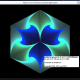# Draw an Apollonian gasket in C#The example Find circles that are tangent to three given circles (Apollonius’ Problem) in C# shows how to find up to eight circles that are tangent to three given circles. This example uses that method to build an Apollonian gasket.

This is also called an Apollonian packing. If you think of each circle as cutting a hole in the enclosing circle so you get a lace-like figure, it’s an Apollonian gasket. If you think of filling the enclosing circle with other circles, it’s a packing.

The following FindApollonianPacking method controls the drawing and returns a Bitmap holding the packing.

```// Find the Apollonian packing.
private Bitmap FindApollonianPacking(int width)
{
Bitmap bm = new Bitmap(width, width);
using (Graphics gr = Graphics.FromImage(bm))
{
gr.SmoothingMode = SmoothingMode.AntiAlias;
gr.Clear(Color.LightGreen);

// Create the three central tangent circles.
float radius = width * 0.225f;
float x = width / 2;
Circle circle0 = new Circle(x, y, radius);

// Draw a box around the gasket. (For debugging.)
//gr.DrawRectangle(Pens.Orange,
//    x - gasket_height / 2,

Circle circle1 = new Circle(x, y, radius);
Circle circle2 = new Circle(x, y, radius);

// Draw the three central circles.
circle0.Draw(gr, Pens.Blue);
circle1.Draw(gr, Pens.Blue);
circle2.Draw(gr, Pens.Blue);

// Find the circle that contains them all.
Circle big_circle = FindApollonianCircle(
circle0, circle1, circle2, -1, -1, -1);
big_circle.Draw(gr, Pens.Blue);

// Set level to smaller values such as 3
// to see partially drawn gaskets.
int level = 10000;

// Find the central circle.
FindCircleOutsideAll(level, gr, circle0, circle1, circle2);

// Find circles tangent to the big circle.
FindCircleOutsideTwo(level, gr,
circle0, circle1, big_circle);
FindCircleOutsideTwo(level, gr,
circle1, circle2, big_circle);
FindCircleOutsideTwo(level, gr,
circle2, circle0, big_circle);
}
return bm;
}```

This method starts by creating a bitmap of the desired size. It then creates the three largest circles inside the enclosing circle. It does a little math to size the circles appropriately, arranges them so they are tangent, and then draws them.

Next the code calls the FindApollonianCircle method used by the previous example to find a circle tangent to the three large circles. It passes the parameters -1, -1, and -1 to find the tangent circle that contains all three of the big circles so the result is the circle that encloses the Apollonian gasket. The program then draws the enclosing circle.

The code then calls the FindCircleOutsideAll method to find and draw the small circle that sits between the three initial circles. It finishes by calling FindCircleOutsideTwo three times to find the circles that lie inside the enclosing circle but outside of two of the inner big circles.

The following code shows the FindCircleOutsideAll method.

```// Draw a circle tangent to these three circles
// and that is outside all three.
private void FindCircleOutsideAll(int level, Graphics gr,
Circle circle0, Circle circle1, Circle circle2)
{
Circle new_circle = FindApollonianCircle(
circle0, circle1, circle2, 1, 1, 1);
if (new_circle == null) return;

new_circle.Draw(gr, Pens.Blue);

if (--level > 0)
{
FindCircleOutsideAll(level, gr,
circle0, circle1, new_circle);
FindCircleOutsideAll(level, gr,
circle0, circle2, new_circle);
FindCircleOutsideAll(level, gr,
circle1, circle2, new_circle);
}
}```

The FindCircleOutsideAll method finds a circle tangent to three other circles that does not contain any of the three. In this program that is the circle that lies between the three other circles. For example, the very center circle lies between the three large circles.

The code calls the FindApollonianCircle method used by the previous example program, passing it the parameters 1, 1, 1 to find the desired circle. If it finds such a circle, the code draws it.

The code then decrements the level parameter and, if it is still greater than 0, the method calls itself recursively three times to find the circles between the new circle and the pairs of the original three circles. (Study the picture to see where those areas are.)

The following code shows the FindCircleOutsideTwo method.

```// Draw a circle tangent to these three circles
// and that is outside two of them.
private void FindCircleOutsideTwo(int level, Graphics gr,
Circle circle0, Circle circle1, Circle circle_contains)
{
Circle new_circle = FindApollonianCircle(
circle0, circle1, circle_contains, 1, 1, -1);
if (new_circle == null) return;

new_circle.Draw(gr, Pens.Blue);

if (--level > 0)
{
FindCircleOutsideTwo(level, gr,
new_circle, circle0, circle_contains);
FindCircleOutsideTwo(level, gr,
new_circle, circle1, circle_contains);
FindCircleOutsideAll(level, gr,
circle0, circle1, new_circle);
}
}```

This method finds a circle that is inside one circle and outside two others. For example, in the figure this method draws the medium-sized circle on the upper right that lies inside the enclosing circle and outside the two big circles.

The code calls the FindApollonianCircle method passing it the parameters 1, 1, and -1 to find the appropriate circle. Note that the order in which the code passes the circles to that method is important because the last circle is the one that should contain the new circle.

Next the code decrements the level parameter and, if it is still greater than 0, the method calls itself recursively three times to find the circles in the areas it has just created. (Again study the picture to see where those areas are.)This entry was posted in algorithms, drawing, graphics, mathematics and tagged , , , , , , , , , , , . Bookmark the permalink.

### 3 Responses to Draw an Apollonian gasket in C#

1.Mason Yu Jr says:

I cant locate the FindApollonianCircle method. Please advise as to where I can locate it.
•RodStephens says: